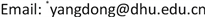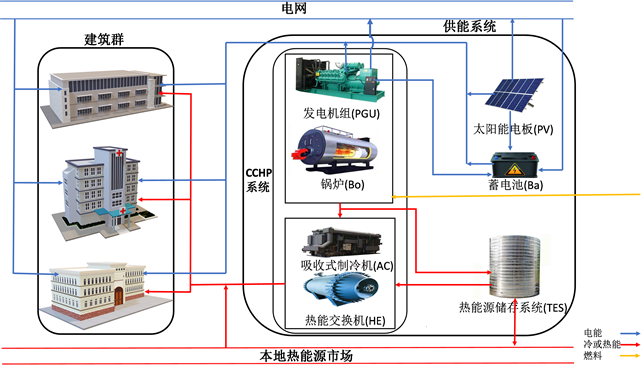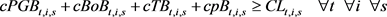﻿ 考虑单个建筑盈利需求的协作式建筑群随机规划决策模型 A Stochastic Decision Model for the Collaborative Building Cluster Considering the Benefit Requirements of Buildings

Management Science and Engineering
Vol. 08  No. 03 ( 2019 ), Article ID: 32260 , 11 pages
10.12677/MSE.2019.83032

A Stochastic Decision Model for the Collaborative Building Cluster Considering the Benefit Requirements of Buildings

Rui Zhou, Jia Li, Dong Yang*, Xiaolin Chu

Glorious Sun School of Business & Management, Donghua University, ShanghaiReceived: Aug. 25th, 2019; accepted: Sep. 13th, 2019; published: Sep. 20th, 2019ABSTRACT

The building cluster’s operation is an important contributor to better energy efficiency and lower energy cost. Yet, the existing research only emphasizes the optimization of the entire building cluster’s collective benefit and neglects the benefit requirements of buildings in the cluster. This will curb the operation of the building cluster when buildings belong to different owners as such owners are profit-seeking. To handle this problem, this paper considers the benefit requirements from buildings, that is, building owners require that the operation of the building cluster provide at least a certain amount of operational cost savings. Otherwise, they would refuse to join in the operation of the building cluster. Additionally, this paper also takes into account the uncertainty in building energy demands. To study the operation of the building cluster with random energy loads considering the benefit requirement from buildings, this research adopts scenario-based stochastic programming to formulate a stochastic mixed-integer programming model. In this model, buildings’ benefit requirements are expressed as constraints. Numerical experimental results demonstrate that, compared to the collaborative operation of the building cluster without buildings’ benefit requirements, the one considering such requirements can provide building owners with the operational cost saves required by them, such as 15% of cost savings, and does not increase the total operational cost of the building cluster, which is still about 31% savings of cost.

Keywords:Smart Building, Random Energy Demand, Stochastic Programming, Benefit Requirement1. 导言

2. 问题描述Figure 1. System architecture for the collaborative building cluster

3. 数学模型

$\mathrm{min}{H}_{total}=\underset{i=1}{\overset{N}{\sum }}{H}_{i}$ (1)

$\begin{array}{c}{H}_{i}=\underset{s=1}{\overset{S}{\sum }}\text{ }P{r}_{s}\underset{t=1}{\overset{T}{\sum }}\left\{{P}_{fPG}fP{G}_{t,i,s}+{P}_{fBo}fB{o}_{t,i,s}+{P}_{pe}\left(ep{B}_{t,i,s}+ep{b}_{t,i,s}\right)\\ \text{\hspace{0.17em}}\text{\hspace{0.17em}}+{P}_{pc}\left(cp{B}_{t,i,s}+cp{T}_{t,i,s}\right)+{P}_{ph}\left(hp{B}_{t,i,s}+hp{T}_{t,i,s}\right)\\ \text{\hspace{0.17em}}\text{\hspace{0.17em}}-{P}_{se}\left(esP{V}_{t,i,s}+esP{G}_{t,i,s}\right)-{P}_{sc}cs{T}_{t,i,s}-{P}_{sh}hs{T}_{t,i,s}\right\}\end{array}$ (2)

${H}_{i}\le Hre{f}_{i}\cdot \left(1-{\stackrel{^}{\gamma }}_{i}\right)\text{\hspace{0.17em}}\text{\hspace{0.17em}}\text{\hspace{0.17em}}\forall i$ (3)

$ePG{B}_{t,i,s}+ePV{B}_{t,i,s}+eb{B}_{t,i,s}+ep{B}_{t,i,s}\ge E{L}_{t,i,s}\text{\hspace{0.17em}}\text{\hspace{0.17em}}\text{\hspace{0.17em}}\forall t\text{\hspace{0.17em}}\text{\hspace{0.17em}}\forall i\text{\hspace{0.17em}}\text{\hspace{0.17em}}\forall s$ (4)(5)

$hPG{B}_{t,i,s}+hBo{B}_{t,i,s}+hT{B}_{t,i,s}+hp{B}_{t,i,s}\ge H{L}_{t,i,s}\text{\hspace{0.17em}}\text{\hspace{0.17em}}\text{\hspace{0.17em}}\forall t\text{\hspace{0.17em}}\text{\hspace{0.17em}}\forall i\text{\hspace{0.17em}}\text{\hspace{0.17em}}\forall s$ (6)

Table 1. Nomenclature for parameters

$\underset{i=1}{\overset{N}{\sum }}\left(ePV{B}_{t,i,s}+ePV{b}_{t,i,s}+esP{V}_{t,i,s}\right)\le SPV\cdot SO{L}_{t}\cdot {\beta }_{PVe}\cdot sP{V}_{t}\text{\hspace{0.17em}}\text{\hspace{0.17em}}\text{\hspace{0.17em}}\forall t\text{\hspace{0.17em}}\text{\hspace{0.17em}}\forall s$ (7)

$\underset{i=1}{\overset{N}{\sum }}\text{ }fP{G}_{t,i,s}\le sP{G}_{t}\cdot SPG\text{\hspace{0.17em}}\text{\hspace{0.17em}}\text{\hspace{0.17em}}\forall t\text{\hspace{0.17em}}\text{\hspace{0.17em}}\forall s$ (8)

$ePG{B}_{t,i,s}+ePG{b}_{t,i,s}+esP{G}_{t,i,s}\le \left(fP{G}_{t,i,s}-{\beta }_{PGe2}\cdot sP{G}_{t,s}\right)/{\beta }_{PGe1}\text{\hspace{0.17em}}\text{\hspace{0.17em}}\text{\hspace{0.17em}}\forall t\text{\hspace{0.17em}}\text{\hspace{0.17em}}\forall i\text{\hspace{0.17em}}\text{\hspace{0.17em}}\forall s$ (9)

$cPG{B}_{t,i,s}+hPG{B}_{t,i,s}+tPG{T}_{t,i,s}\le fP{G}_{t.i,s}\cdot {\beta }_{PGt}\text{\hspace{0.17em}}\text{\hspace{0.17em}}\text{\hspace{0.17em}}\forall t\text{\hspace{0.17em}}\text{\hspace{0.17em}}\forall i\text{\hspace{0.17em}}\text{\hspace{0.17em}}\forall s$ (10)

$\underset{i=1}{\overset{N}{\sum }}\text{ }fB{o}_{t,i,s}\le sB{o}_{t}\cdot SBo\text{\hspace{0.17em}}\text{\hspace{0.17em}}\text{\hspace{0.17em}}\forall t\text{\hspace{0.17em}}\text{\hspace{0.17em}}\forall s$ (11)

$cBo{B}_{t,i,s}+hBo{B}_{t,i,s}+tBo{T}_{t,i,s}\le fB{o}_{t.i,s}\cdot {\beta }_{Bot}\text{\hspace{0.17em}}\text{\hspace{0.17em}}\text{\hspace{0.17em}}\forall t\text{\hspace{0.17em}}\text{\hspace{0.17em}}\forall i\text{\hspace{0.17em}}\text{\hspace{0.17em}}\forall s$ (12)

$sb{c}_{t}+sb{d}_{t}\le 1\text{\hspace{0.17em}}\text{\hspace{0.17em}}\text{\hspace{0.17em}}\forall t$ (13)

$E{b}_{\mathrm{min}}\le e{b}_{t,s}\le E{b}_{\mathrm{max}}\text{\hspace{0.17em}}\text{\hspace{0.17em}}\text{\hspace{0.17em}}\forall t\text{\hspace{0.17em}}\text{\hspace{0.17em}}\forall s$ (14)

$sb{c}_{t}\cdot Eb{c}_{\mathrm{min}}\le eb{c}_{t,s}\le sb{c}_{t}\cdot Eb{c}_{\mathrm{max}}\text{\hspace{0.17em}}\text{\hspace{0.17em}}\text{\hspace{0.17em}}\forall t\text{\hspace{0.17em}}\text{\hspace{0.17em}}\forall s$ (15)

$sb{d}_{t}\cdot Eb{d}_{\mathrm{min}}\le eb{d}_{t,s}\le sb{d}_{t}\cdot Eb{d}_{\mathrm{max}}\text{\hspace{0.17em}}\text{\hspace{0.17em}}\text{\hspace{0.17em}}\forall t\text{\hspace{0.17em}}\text{\hspace{0.17em}}\forall s$ (16)

$eb{c}_{t,s}=\underset{i=1}{\overset{N}{\sum }}\left(ePG{b}_{t,i,s}+ePV{b}_{t,i,s}+eP{b}_{t,i,s}\right)\cdot {\beta }_{bc}\text{\hspace{0.17em}}\text{\hspace{0.17em}}\text{\hspace{0.17em}}\forall t\text{\hspace{0.17em}}\text{\hspace{0.17em}}\forall s$ (17)

$eb{d}_{t,s}=\underset{i=1}{\overset{N}{\sum }}\text{ }eb{B}_{t,i,s}/{\beta }_{bd}\text{\hspace{0.17em}}\text{\hspace{0.17em}}\text{\hspace{0.17em}}\forall t\text{\hspace{0.17em}}\text{\hspace{0.17em}}\forall s$ (18)

$e{b}_{1,s}=e{b}_{0}+\left(eb{c}_{1,s}-eb{d}_{1,s}\right)\cdot \Delta t\text{\hspace{0.17em}}\text{\hspace{0.17em}}\text{\hspace{0.17em}}\text{\hspace{0.17em}}\forall s$ (19)

$e{b}_{t,s}=e{b}_{t-1,s}+\left(eb{c}_{t,s}-eb{d}_{t,s}\right)\cdot \Delta t\text{\hspace{0.17em}}\text{\hspace{0.17em}}\text{\hspace{0.17em}}\forall t\ge 2\text{\hspace{0.17em}}\text{\hspace{0.17em}}\forall s$ (20)

$sT{c}_{t}+sT{d}_{t}\le 1\text{\hspace{0.17em}}\text{\hspace{0.17em}}\text{\hspace{0.17em}}\forall t$ (21)

$T{T}_{\mathrm{min}}\le t{T}_{t,s}\le T{T}_{\mathrm{max}}\text{\hspace{0.17em}}\text{\hspace{0.17em}}\text{\hspace{0.17em}}\forall t\text{\hspace{0.17em}}\text{\hspace{0.17em}}\forall s$ (22)

$sT{c}_{t}\cdot TT{c}_{\mathrm{min}}\le tT{c}_{t,s}\le sT{c}_{t}\cdot TT{c}_{\mathrm{max}}\text{\hspace{0.17em}}\text{\hspace{0.17em}}\text{\hspace{0.17em}}\forall t\text{\hspace{0.17em}}\text{\hspace{0.17em}}\forall s$ (23)

$sT{d}_{t}\cdot TT{d}_{\mathrm{min}}\le tT{d}_{t,s}\le sT{d}_{t}\cdot TT{d}_{\mathrm{max}}\text{\hspace{0.17em}}\text{\hspace{0.17em}}\text{\hspace{0.17em}}\forall t\text{\hspace{0.17em}}\text{\hspace{0.17em}}\forall s$ (24)

$tT{c}_{t,s}=\underset{i=1}{\overset{N}{\sum }}\left(tPG{T}_{t,i,s}+tBo{T}_{t,i,s}+hp{T}_{t,i,s}+cp{T}_{t,i,s}\right)\cdot {\beta }_{Tc}\text{\hspace{0.17em}}\text{\hspace{0.17em}}\text{\hspace{0.17em}}\forall t\text{\hspace{0.17em}}\text{\hspace{0.17em}}\forall s$ (25)

$tT{d}_{t,s}=\underset{i=1}{\overset{N}{\sum }}\left(hT{B}_{t,i,s}+hs{T}_{t,i,s}+cT{B}_{t,i,s}+cs{T}_{t,i,s}\right)/{\beta }_{Td}\text{\hspace{0.17em}}\text{\hspace{0.17em}}\text{\hspace{0.17em}}\forall t\text{\hspace{0.17em}}\text{\hspace{0.17em}}\forall s$ (26)

$t{T}_{1,s}=t{T}_{0}+\left(tT{c}_{1,s}-tT{d}_{1,s}\right)\cdot \Delta t\text{\hspace{0.17em}}\text{\hspace{0.17em}}\text{\hspace{0.17em}}\forall s$ (27)

$t{T}_{t,s}=t{T}_{t-1,s}+\left(tT{c}_{t,s}-tT{d}_{t,s}\right)\cdot \Delta t\text{\hspace{0.17em}}\text{\hspace{0.17em}}\text{\hspace{0.17em}}\text{\hspace{0.17em}}\forall t\ge 2\text{\hspace{0.17em}}\text{\hspace{0.17em}}\forall s$ (28)

$\underset{i=1}{\overset{N}{\sum }}\left(ep{B}_{t,i,s}+ep{b}_{t,i,s}\right)\le SeG\text{\hspace{0.17em}}\text{\hspace{0.17em}}\text{\hspace{0.17em}}\forall t\text{\hspace{0.17em}}\text{\hspace{0.17em}}\forall s$ (29)

$\underset{i=1}{\overset{N}{\sum }}\left(esP{V}_{t,i,s}+esP{G}_{t,i,s}\right)\le SeG\text{\hspace{0.17em}}\text{\hspace{0.17em}}\text{\hspace{0.17em}}\forall t\text{\hspace{0.17em}}\text{\hspace{0.17em}}\forall s$ (30)

$\underset{i=1}{\overset{N}{\sum }}\left(cp{B}_{t,i,s}+cp{T}_{t,i,s}+hp{B}_{t,i,s}+hp{T}_{t,i,s}\right)\le StL\text{\hspace{0.17em}}\text{\hspace{0.17em}}\text{\hspace{0.17em}}\forall t\text{\hspace{0.17em}}\text{\hspace{0.17em}}\forall s$ (31)

$\underset{i=1}{\overset{N}{\sum }}\left(cs{T}_{t,i,s}+hs{T}_{t,i,s}\right)\le StL\text{\hspace{0.17em}}\text{\hspace{0.17em}}\text{\hspace{0.17em}}\forall t\text{\hspace{0.17em}}\text{\hspace{0.17em}}\forall s$ (32)

4. 算例实验结果及其分析

4.1. 算例数据Table 3. Comparison between the operation of the building cluster considering the benefit requirements of buildings and the one without the benefit requirements of buildings

5. 结论

A Stochastic Decision Model for the Collaborative Building Cluster Considering the Benefit Requirements of Buildings[J]. 管理科学与工程, 2019, 08(03): 264-274. https://doi.org/10.12677/MSE.2019.83032

1. 1. Pérez-Lombard, L., Ortiz, J. and Pout, C. (2008) A Review on Buildings Energy Consumption Information. Energy and Buildings, 40, 394-398.
https://doi.org/10.1016/j.enbuild.2007.03.007

2. 2. US Department of Energy, Energy Information Administration (2016) International Energy Outlook.
https://www.eia.gov/outlooks/ieo/

3. 3. Hu, M., Weir, J.D. and Wu, T. (2012) Decentralized Operation Strategies for an Inte-grated Building Energy System Using a Memetic Algorithm. European Journal of Operational Research, 217, 185-197.
https://doi.org/10.1016/j.ejor.2011.09.008

4. 4. Jafari-Marandi, R., Hu, M. and Omitaomu, O.A. (2016) A Distributed Decision Framework for Building Clusters with Different Heterogeneity Settings. Applied Energy, 165, 393-404.
https://doi.org/10.1016/j.apenergy.2015.12.088

5. 5. Vigna, I., Pernetti, R., Pasut, W. and Lollini, R. (2018) New Domain for Promoting Energy Efficiency: Energy Flexible Building Cluster. Sustainable Cities and Society, 38, 526-533.
https://doi.org/10.1016/j.scs.2018.01.038

6. 6. Dai, R., Hu, M., Yang, D. and Chen, Y. (2015) A Collaborative Operation Deci-sion Model for Distributed Building Clusters. Energy, 84, 759-773.
https://doi.org/10.1016/j.energy.2015.03.042

7. 7. Huang, P. and Sun, Y. (2019) A Collaborative Demand Control of Nearly Zero Energy Buildings in Response to Dynamic Pricing for Per-formance Improvements at Cluster Level. Energy, 174, 911-921.
https://doi.org/10.1016/j.energy.2019.02.192

8. 8. Huang, P., Wu, H., Huang, G. and Sun, Y. (2018) A Top-Down Control Method of nZEBs for Performance Optimization at nZEB-Cluster-Level. Energy, 159, 891-904.
https://doi.org/10.1016/j.energy.2018.06.199

9. 9. Huang, P., Fan, C., Zhang, X. and Wang, J. (2019) A Hierarchical Coordi-nated Demand Response Control for Buildings with Improved Performances at Building Group. Applied Energy, 242, 684-694.
https://doi.org/10.1016/j.apenergy.2019.03.148

10. 10. 曼昆NG. 经济学原理[M]. 北京: 机械工业出版社, 2003.

11. 11. Hu, M. (2015) A Data-Driven Feed-Forward Decision Framework for Building Clusters Operation under Uncertainty. Applied Energy, 141, 229-237.
https://doi.org/10.1016/j.apenergy.2014.12.047

12. 12. Wahui, T., Sawada, K., Kawayoshi, H., Yokoyama, R., Litaka, H. and Aki, H. (2017) Optimal Operations Management of Residential Energy Supply Networks with Power and Heat Interchanges. Energy and Buildings, 151, 167-186.
https://doi.org/10.1016/j.enbuild.2017.06.041

13. 13. Birge, J.R. and Louveaux, F.V. (2011) Introduction to Stochastic Program-ming. 2rd Edition, Springer, New York.
https://doi.org/10.1007/978-1-4614-0237-4

14. 14. Mehleri, E.D., Sarimveis, H., Markatos, N.C. and Papageorgious, L.G. (2012) A Mathematical Programming Approach for Optimal Design of Distributed Energy Systems at the Neighbourhood Level. Energy, 44, 96-104.
https://doi.org/10.1016/j.energy.2012.02.009

15. 15. National Renewable Energy Laboratory (2019) National Solar Radiation Data Base. http://rredc.nrel.gov/solar/old_data/nsrdb/

16. 16. Tan, Z., Zhang, H., Shi, Q., Song, Y. and Ju, L. (2014) Multi-Objective Operation and Evaluation of Large-Scale NG Distributed Energy System Driven by Gas-Steam Combined Cycle in China. Energy and Buildings, 76, 572-587.
https://doi.org/10.1016/j.enbuild.2014.03.029

17. 17. Cho, H., Mago, P., Luck, R. and Chamra, L. (2009) Evaluation of CCHP Systems Performance Based on Operational Cost, Primary Energy Consumption, and Carbon Dioxide Emission by Utilizing an Op-timal Operation Scheme. Applied Energy, 86, 2540-2549.
https://doi.org/10.1016/j.apenergy.2009.04.012

18. 18. Zhou, Z., Zhang, J., Liu, P., Li, Z., Georgiadis, M.C. and Pistikopoulos, E.N. (2013) A Two-Stage Stochastic Programming Model for the Optimal Design of Distributed Energy Systems. Applied Energy, 103, 135-144.
https://doi.org/10.1016/j.apenergy.2012.09.019

$\mathrm{min}Hre{f}_{i}$ (33)

$sP{V}_{t}=sP{G}_{t}=sB{o}_{t}=sb{c}_{t}=sb{d}_{t}=sT{c}_{t}=sT{d}_{t}=0\text{\hspace{0.17em}}\text{\hspace{0.17em}}\text{\hspace{0.17em}}\forall t$ (34)

NOTES

*通讯作者。

1Detailed information of SPR electricity price is available at https://www.srpnet.com/.